Study Materials: ncert solutions

Our ncert solutions for Exercise 10.1 Class 11 maths 10. Straight Lines - ncert solutions - Toppers Study is the best material for English Medium students cbse board and other state boards students.

Solutions ⇒ Class 11th ⇒ Mathematics ⇒ 10. Straight Lines

# Exercise 10.1 Class 11 maths 10. Straight Lines - ncert solutions - Toppers Study

Topper Study classes prepares ncert solutions on practical base problems and comes out with the best result that helps the students and teachers as well as tutors and so many ecademic coaching classes that they need in practical life. Our ncert solutions for Exercise 10.1 Class 11 maths 10. Straight Lines - ncert solutions - Toppers Study is the best material for English Medium students cbse board and other state boards students.

## Exercise 10.1 Class 11 maths 10. Straight Lines - ncert solutions - Toppers Study

CBSE board students who preparing for class 11 ncert solutions maths and Mathematics solved exercise chapter 10. Straight Lines available and this helps in upcoming exams 2023-2024.

### You can Find Mathematics solution Class 11 Chapter 10. Straight Lines

• All Chapter review quick revision notes for chapter 10. Straight Lines Class 11
• NCERT Solutions And Textual questions Answers Class 11 Mathematics
• Extra NCERT Book questions Answers Class 11 Mathematics
• Importatnt key points with additional Assignment and questions bank solved.

NCERT Solutions do not only help you to cover your syllabus but also will give to textual support in exams 2023-2024 to complete exercise 10.1 maths class 11 chapter 10 in english medium. So revise and practice these all cbse study materials like class 11 maths chapter 10.1 in english ncert book. Also ensure to repractice all syllabus within time or before board exams for ncert class 11 maths ex 10.1 in english.

See all solutions for class 11 maths chapter 10 exercise 10 in english medium solved questions with answers.

### Exercise 10.1 class 11 Mathematics Chapter 10. Straight Lines

• Exercise 10.1 Class 11 Maths 10. Straight Lines - Ncert Solutions - Toppers Study
• Class 11 Ncert Solutions
• Solution Chapter 10. Straight Lines Class 11
• Solutions Class 11
• Chapter 10. Straight Lines Exercise 10.1 Class 11

## Exercise 10.1 Class 11 maths 10. Straight Lines - ncert solutions - Toppers Study

Exercise 10.1

Q1. Draw a quadrilateral in the Cartesian plane, whose vertices are (– 4, 5), (0, 7), (5, – 5) and (– 4, –2). Also, find its area.Solution: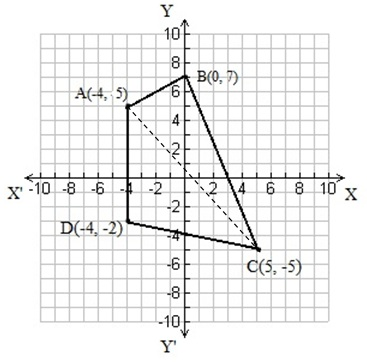Let ABCD be the given quadrilateral with vertices A (–4, 5), B (0, 7), C (5, –5), and D (– 4, –2).

Now, by plotting A, B, C, and D on the Cartesian plane and joining AB, BC, CD, and DA, the given quadrilateral can be drawn as joining Point A to C forms a diagonal AC.

Accordingly, area (ABCD) = area(∆ABC) + area (∆ACD)

Using area of triangle formula.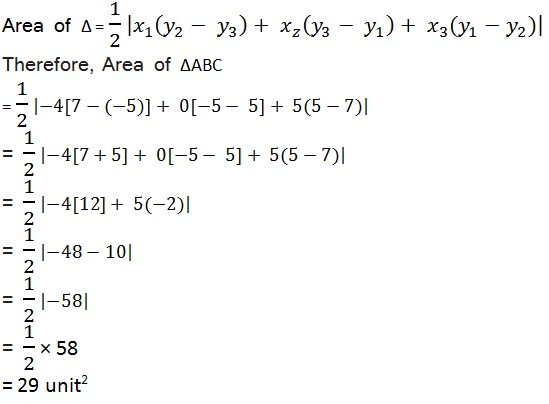Therefore, Area of ∆ACD where A (-4, 5), C (5, -5), D (-4, -2)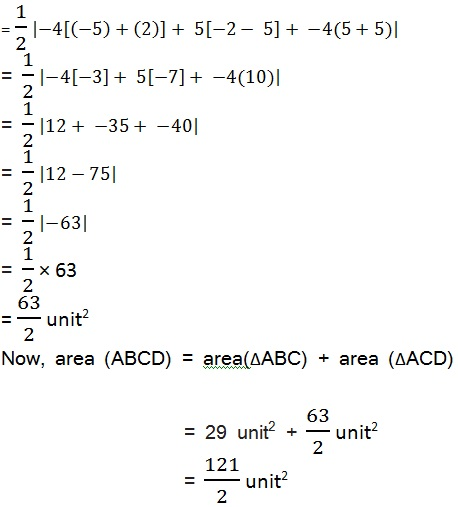Q2. The base of an equilateral triangle with side 2a lies along the y-axis such that the mid-point of the base is at the origin. Find vertices of the triangle.

Solution: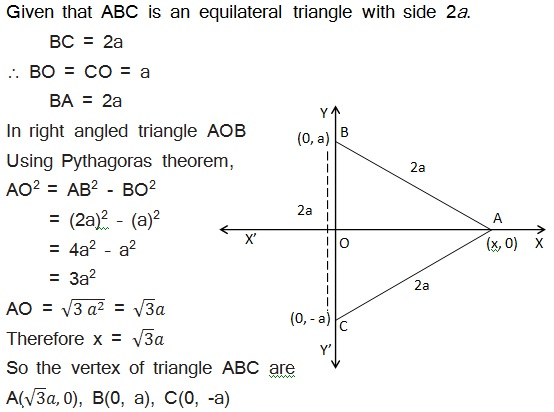Q3. Find the dis tance between P (x1, y1) and Q (x2, y2) when: (i) PQ is parallel to the y-axis, (ii) PQ is parallel to the x-axis.

Solution:

Given points are : P(x1, y1) and Q(x2, y2)

(i) When PQ is parallel to the y-axis then x1 = x2

Using Distance formula for distance between P and Q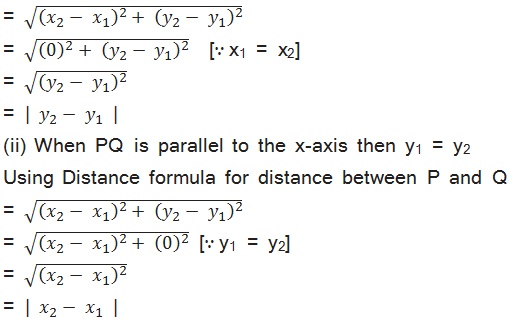Q4. Find a point on the x-axis, which is equidistant from the points (7, 6) and (3, 4).

Solution:

Let the point on x-axis be P (x, 0) which is equidistance from points A (7, 6) and B (3, 4).

Accordingly,

AP = BP

Or    AP2 = BP2  [Squaring both sides]

(x – 7)2 + (0 – 6)2 = (x – 3)2 + (0 – 4)2

x2 – 14x + 49 + 36 = x2 – 6x + 9 + 16

x2 – 14x + 85 = x2 – 6x + 25

85 – 25 = 14x – 6x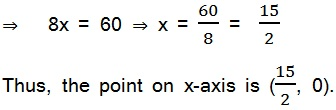Q5. Find the slope of a line, which passes through the origin and the mid-point of the line segment joining the points P (0, 4) and B (8, 0).

Solution:

The coordinates of the mid-point of the line segment joining the points P (0, 4) and B (8, 0).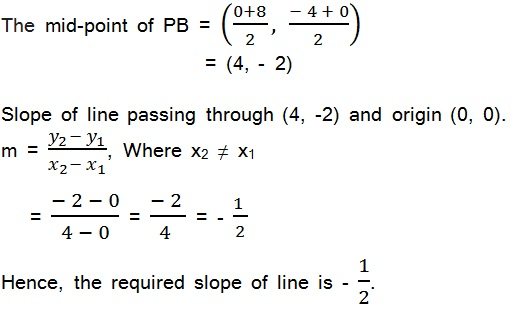Q6. Without using the Pythagoras theorem, show that the points (4, 4), (3, 5) and (1, 1) are the vertices of a right angled triangle.Solution:

The vertices of the given triangle are

A (4, 4), B (3, 5), and C (–1, –1).

If given vertices are of a right angle triangle.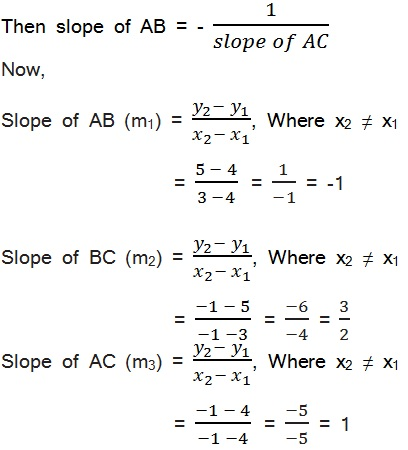m1m3 = -1

Slope of AB (m1) × Slope of AC (m3) = -1

It means side AB and AC are perpendicular to each other.

Here, given triangle is right-angled at point A (4, 4).

Thus, the points (4, 4), (3, 5), and (–1, –1) are the vertices of a right-angled triangle.

Q7. Find the slope of the line, which makes an angle of 30° with the positive direction of y-axis measured anticlockwise.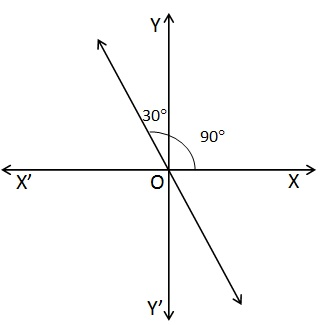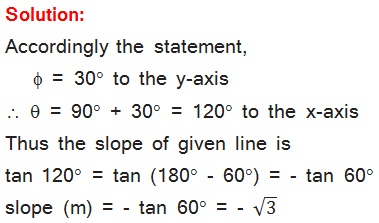Q8. Find the value of x for which the points (x, – 1), (2,1) and (4, 5) are collinear.

Solution:

Let point be A (x, –1), B (2, 1), and C (4, 5).

If points A (x, –1), B (2, 1), and C (4, 5) are collinear, then

Slope of AB = Slope of BC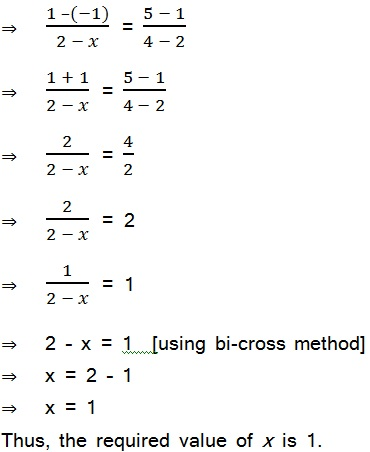Q9. Without using distance formula, show that points (2, 1), (4, 0), (3, 3) and (3, 2) are the vertices of a parallelogram.

Solution:

To be the points A(2, 1), B(4, 0), C(3, 3) and D(3, 2) of the vertices of a parallelogram.

There must be AB || CD or BC || AD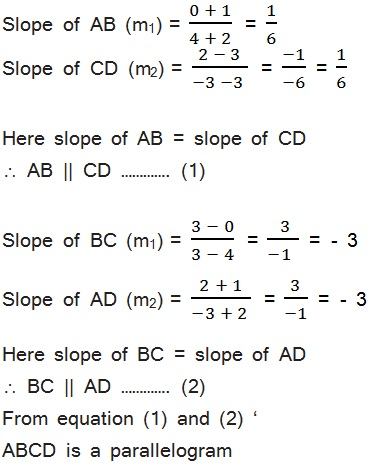Thus, points (–2, –1), (4, 0), (3, 3), and (–3, 2) are the vertices of a parallelogram.

Q10. Find the angle between the x-axis and the line joining the points (3,1) and (4,2).

Solution:

Let be points A(3,1) and B(4,2) are given for a line.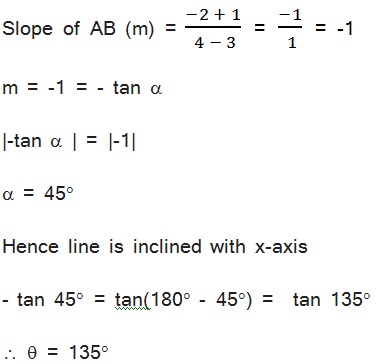Thus, the angle between the x-axis and the line joining the points (3, –1) and (4, –2) is 135°.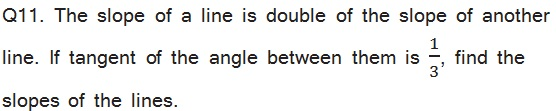Solution: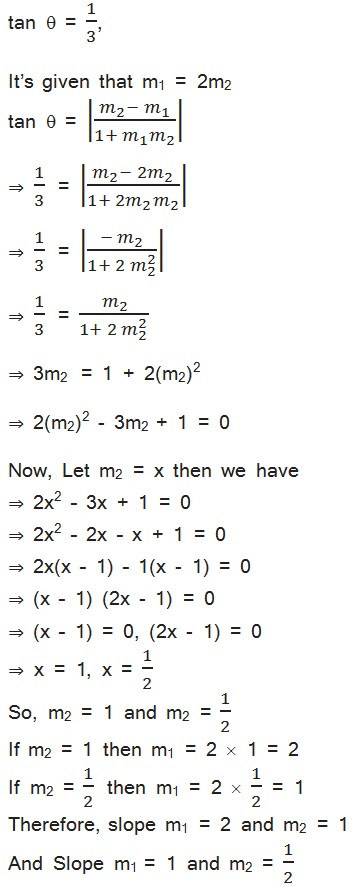Q12. A line passes through (x1, y1) and (h, k). If slope of the line is m, show that k – y1 = m (h – x1).

Solution:

Line passes through points (x1, y1) and (h, k).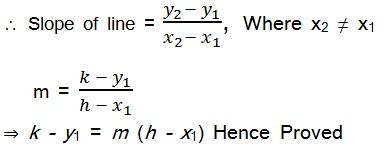Q14. Consider the following population and year graph, find the slope of the line AB and using it, find what will be the population in the year 2010?Solution:

Line AB passes through points A(1985, 92) and B(1995, 97).Let y be the population in the year 2010. Then, according to the given graph, line AB must pass through point C (2010, y).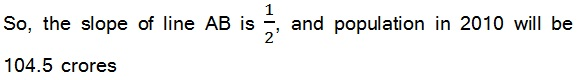##### Other Pages of this Chapter: 10. Straight Lines

Important Study materials for classes 06, 07, 08,09,10, 11 and 12. Like CBSE Notes, Notes for Science, Notes for maths, Notes for Social Science, Notes for Accountancy, Notes for Economics, Notes for political Science, Noes for History, Notes For Bussiness Study, Physical Educations, Sample Papers, Test Papers, Mock Test Papers, Support Materials and Books.Mathematics Class - 11th

NCERT Maths book for CBSE Students.

books

## Study Materials List:

##### Solutions ⇒ Class 11th ⇒ Mathematics
1. Sets
2. Relations and Functions
3. Trigonometric Functions
4. Principle Of Mathematical Induction
5. Complex Numbers and Quadratic Equations
6. Linear Inequalities
7. Permutations and Combinations
8. Binomial Theorem
9. Sequences and Series
10. Straight Lines
11. Conic Sections
12. Introduction to Three Dimensional Geometry
13. Limits and Derivatives
14. Mathematical Reasoning
15. Statistics
16. Probability

New Books